Electron. J. Differential Equations, Vol. 2016 (2016), No. 339, pp. 1-18.

### Infinitely many solutions for Schrodinger-Kirchhoff type equations involving the fractional p-Laplacian and critical exponent Li Wang, Binlin Zhang

Abstract:
In this article, we show the existence of infinitely many solutions for the fractional p-Laplacian equations of Schrodinger-Kirchhoff type equation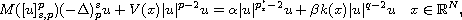where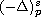is the fractional p-Laplacian operator,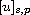is the Gagliardo p-seminorm,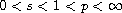,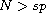,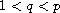, M is a continuous and positive function, V is a continuous and positive potential function and k(x) is a non-negative function in an appropriate Lebesgue space. By means of the concentration-compactness principle in fractional Sobolev space and Kajikiya's new version of the symmetric mountain pass lemma, we obtain the existence of infinitely many solutions which tend to zero for suitable positive parametersand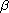.

Submitted October 11, 2016. Published December 30, 2016.
Math Subject Classifications: 35R11, 35A15, 47G20.
Key Words: Schrodinger-Kirchhoff type equation; fractional p-Laplacian; critical Sobolev exponent.

Show me the PDF file (308 KB), TEX file for this article.Li Wang School of Basic Science East China Jiaotong University Nanchang 330013, China email: wangli.423@163.com Binlin Zhang Department of Mathematics Heilongjiang Institute of Technology Harbin 150050, China email: zhangbinlin2012@163.com